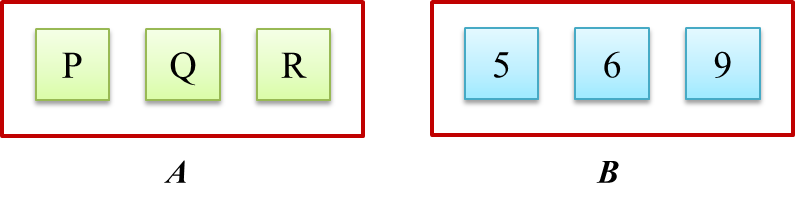# SPM Mathematics (Model Test Paper), Question 10 & 11

SPM Mathematics (Model Test Paper)

Section A
(52 marks)
Answer all questions in this section.

10.   Diagram 7.1 shows three cards labelled with letters in bag A and three numbered cards in bag B.Diagram 7.1

A card is picked at random from bag A and then a card is picked at random from bag B.

(a) Diagram 7.2 in the answer space shows the incomplete possible outcomes of the  event.
Complete the possible outcomes in Diagram 7.2.

(b)   Using the complete possible outcomes in 10(a), find the probability that
(i) a card labelled Q and the card with odd number are picked,
(ii)   a card labelled P or the card with a number which is multiple of 3 are picked.
[5 marks]
(a)Diagram 7.2

(a)(b)(i)
n (s) = 9
P (a card labelled Q and a card with odd number)
= {(Q 5), (Q 9)}
$=\frac{2}{9}$

(b)(ii)
P (a card labelled P or a card with a number which is multiple of 3)
= {(P 5), (P 6), (P 9), (Q 6), (Q 9), (R 6), (R 9)}
$=\frac{7}{9}$

11.   It is given that matrix M is a 2 × 2 matrix such that
(a) Find matrix M.
(b)   Write the following simultaneous linear equations as matrix equations:
–2x + y = 10
x + 3= 9
Hence, using matrix method, calculate the value of x and of y. [6 marks]

$\begin{array}{l}\end{array}$